Next: Zero-velocity surfaces Up: Three-body problem Previous: Co-rotating frame

# Lagrange points

Let us search for possible equilibrium points of the mass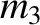in the rotating reference frame. Such points are termed Lagrange points. Hence, in the rotating frame, the masswould remain at rest if placed at one of the Lagrange points. It is, thus, clear that these points are fixed in the rotating frame. Conversely, in the inertial reference frame, the Lagrange points rotate about the center of mass with angular velocity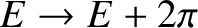, and the masswould consequently also rotate about the center of mass with angular velocityif placed at one of these points (with the appropriate velocity). In the following, we shall assume, without loss of generality, that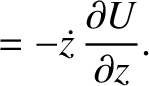.

The Lagrange points satisfy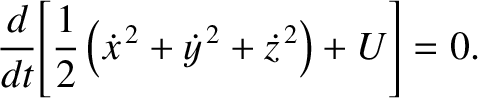in the rotating frame. It thus follows, from Equations (9.27)-(9.29), that the Lagrange points are the solutions of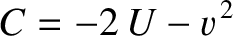(9.37)

It is easily seen that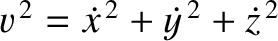(9.38)

Because the term in brackets is positive definite, we conclude that the only solution to the preceding equation is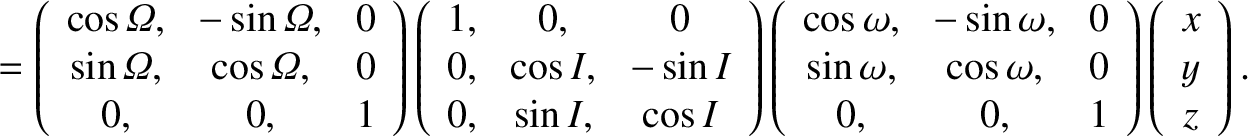. Hence, all of the Lagrange points lie in the-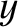plane.

If, it is readily demonstrated that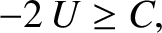(9.39)

where use has been made of the fact that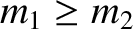. Hence, Equation (9.30) can also be written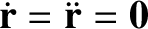(9.40)

The Lagrange points thus satisfy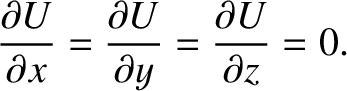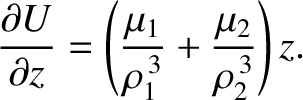(9.41) and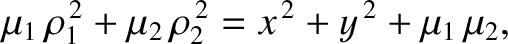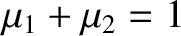(9.42)

which reduce to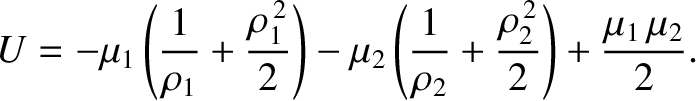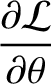(9.43) and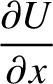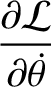(9.44)

One obvious solution of Equation (9.44) is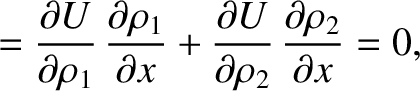, corresponding to a Lagrange point that lies on the-axis. It turns out that there are three such points: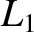lies between masses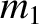and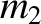,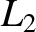lies to the right of mass, and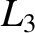lies to the left of mass. (See Figure 9.6.) At thepoint, we have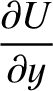and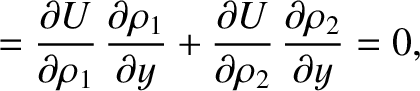. Hence, from Equation (9.43),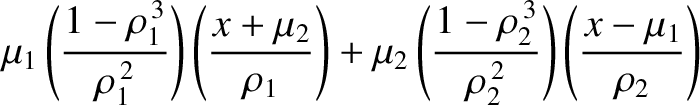(9.45)

Assuming that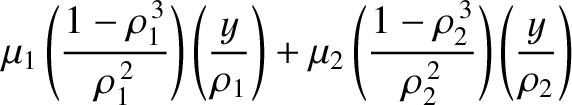, we can find an approximate solution of Equation (9.45) by expanding in powers of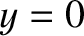: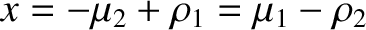(9.46)

This equation can be inverted to give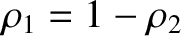(9.47)

where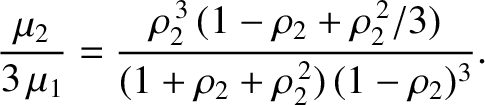(9.48)

is assumed to be a small parameter.

At thepoint, we have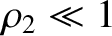and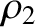. Hence, from Equation (9.43),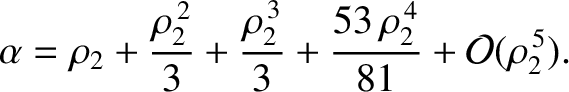(9.49)

Again, expanding in powers of, we obtain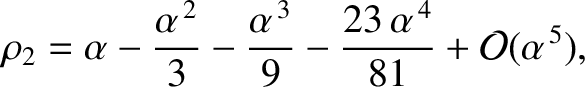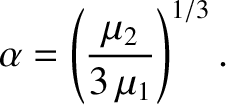(9.50) and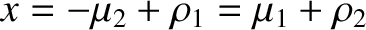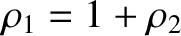(9.51)

Finally, at thepoint, we have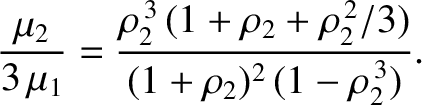and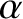. Hence, from Equation (9.43),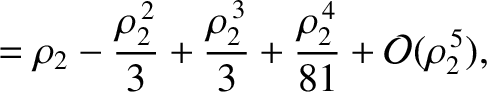(9.52)

Let. Expanding in powers of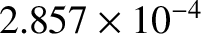, we obtain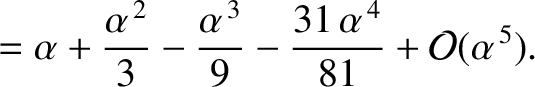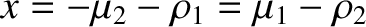(9.53) and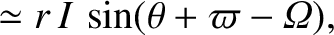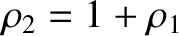(9.54)

where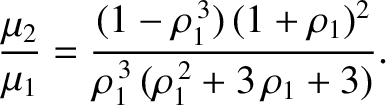is assumed to be a small parameter.

Let us now search for Lagrange points that do not lie on the-axis. One obvious solution of Equations (9.41) and (9.42) is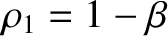(9.55)

giving, from Equation (9.40),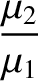(9.56)

or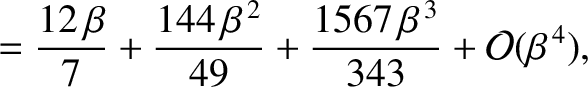(9.57)

because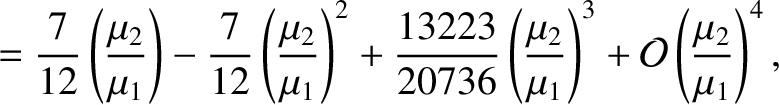. The two solutions of this equation are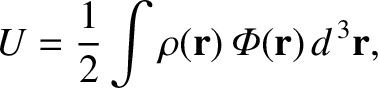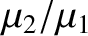(9.58) and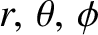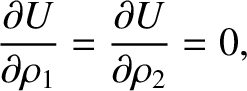(9.59)

and specify the positions of the Lagrange points designated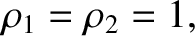and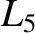. Note that the pointand the massesandlie at the apexes of an equilateral triangle. The same is true for the point. We have now found all of the possible Lagrange points.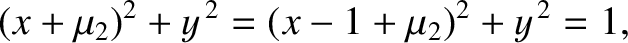Figure 9.6 shows the positions of the two masses,and, and the five Lagrange points,to, calculated for the case where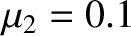.Next: Zero-velocity surfaces Up: Three-body problem Previous: Co-rotating frame
Richard Fitzpatrick 2016-03-31